# Data Flow Analysis Data Flow Analysis n Goal

• Slides: 11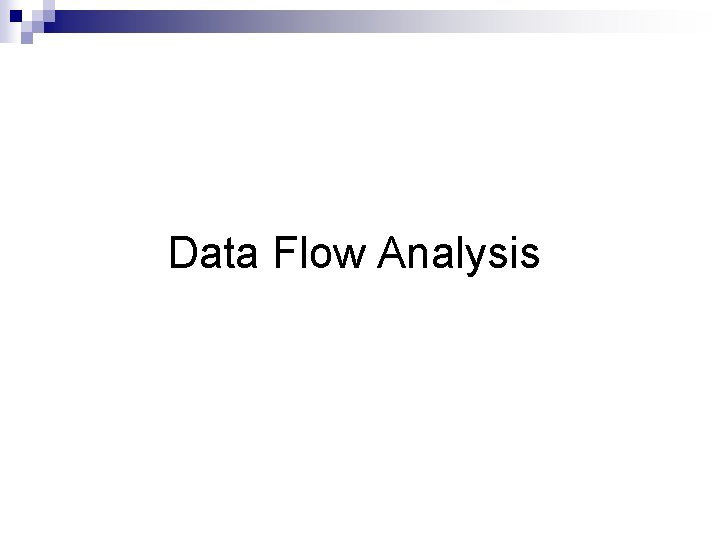Data Flow Analysis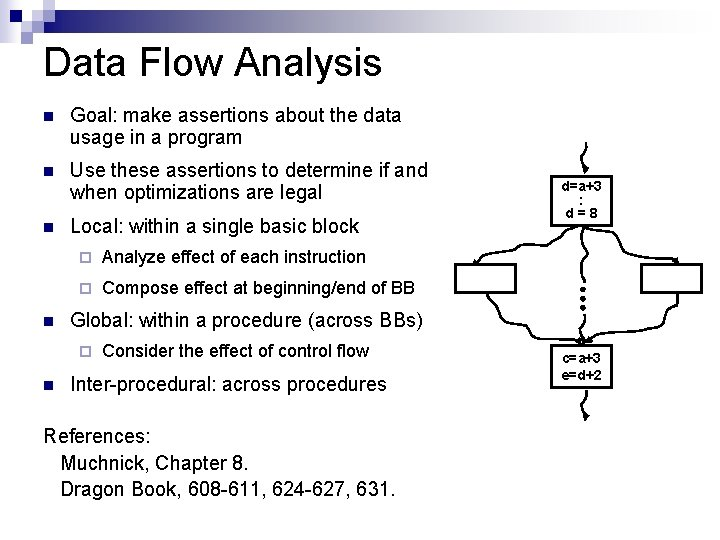Data Flow Analysis n Goal: make assertions about the data usage in a program n Use these assertions to determine if and when optimizations are legal n Local: within a single basic block n ¨ Analyze effect of each instruction ¨ Compose effect at beginning/end of BB Global: within a procedure (across BBs) ¨ n d=a+3 : d=8 Consider the effect of control flow Inter-procedural: across procedures References: Muchnick, Chapter 8. Dragon Book, 608 -611, 624 -627, 631. c=a+3 e=d+2Data Flow Analysis n n Compile-time reasoning about the run-time flow of values in the program Represent facts about the run-time behavior Represent effect of executing each basic block Propagate facts around the control flow graphData Flow Analysis n Formulated as a set of simultaneous equations ¨ Sets attached to the nodes and edges ¨ Lattice to describe the relation between values ¨ Usually represented as a bit or bit vectors n Solve equations using iterative framework ¨ Start with initial guess of facts at each node ¨ Propagate until stabilizes at maximal fixed point. ¨ Would like meet over all paths (MOP) solution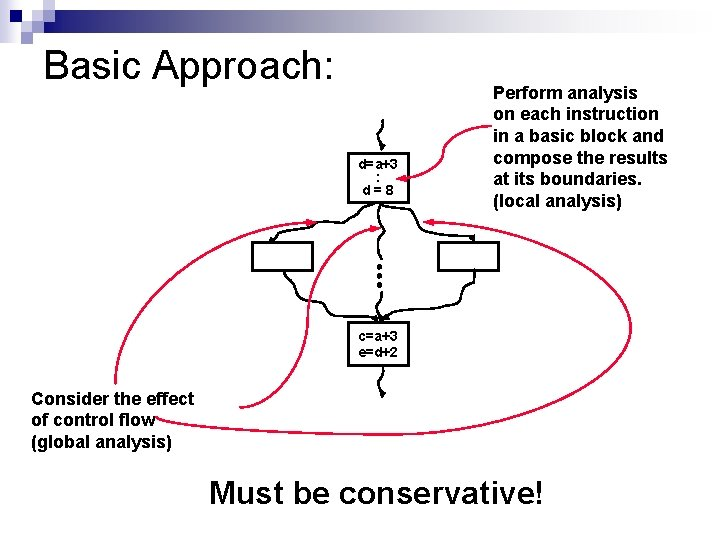Basic Approach: d=a+3 : d=8 Perform analysis on each instruction in a basic block and compose the results at its boundaries. (local analysis) c=a+3 e=d+2 Consider the effect of control flow (global analysis) Must be conservative!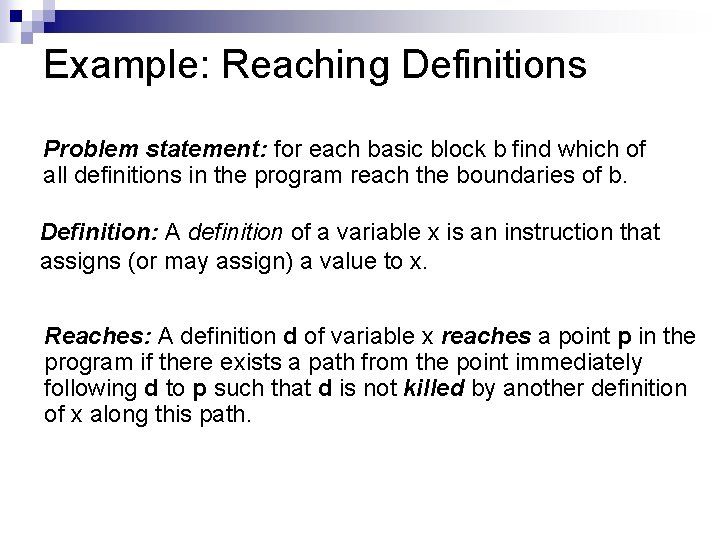Example: Reaching Definitions Problem statement: for each basic block b find which of all definitions in the program reach the boundaries of b. Definition: A definition of a variable x is an instruction that assigns (or may assign) a value to x. Reaches: A definition d of variable x reaches a point p in the program if there exists a path from the point immediately following d to p such that d is not killed by another definition of x along this path.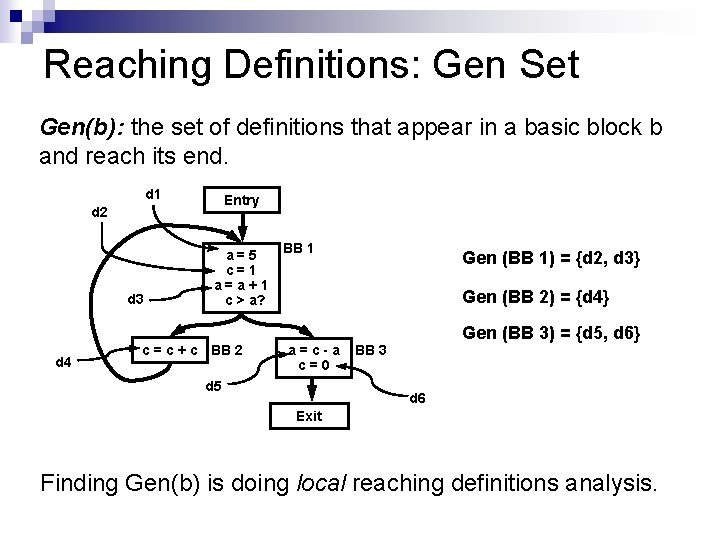Reaching Definitions: Gen Set Gen(b): the set of definitions that appear in a basic block b and reach its end. d 1 Entry d 2 d 3 d 4 a=5 c=1 a=a+1 c > a? c = c + c BB 2 BB 1 Gen (BB 1) = {d 2, d 3} Gen (BB 2) = {d 4} a=c-a c=0 d 5 Gen (BB 3) = {d 5, d 6} BB 3 d 6 Exit Finding Gen(b) is doing local reaching definitions analysis.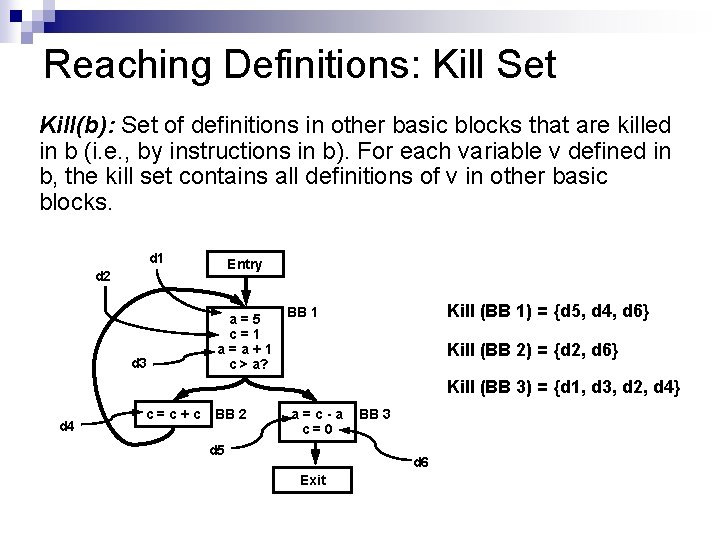Reaching Definitions: Kill Set Kill(b): Set of definitions in other basic blocks that are killed in b (i. e. , by instructions in b). For each variable v defined in b, the kill set contains all definitions of v in other basic blocks. d 1 Entry d 2 d 3 a=5 c=1 a=a+1 c > a? Kill (BB 1) = {d 5, d 4, d 6} BB 1 Kill (BB 2) = {d 2, d 6} Kill (BB 3) = {d 1, d 3, d 2, d 4} d 4 c = c + c BB 2 a=c-a c=0 d 5 BB 3 d 6 Exit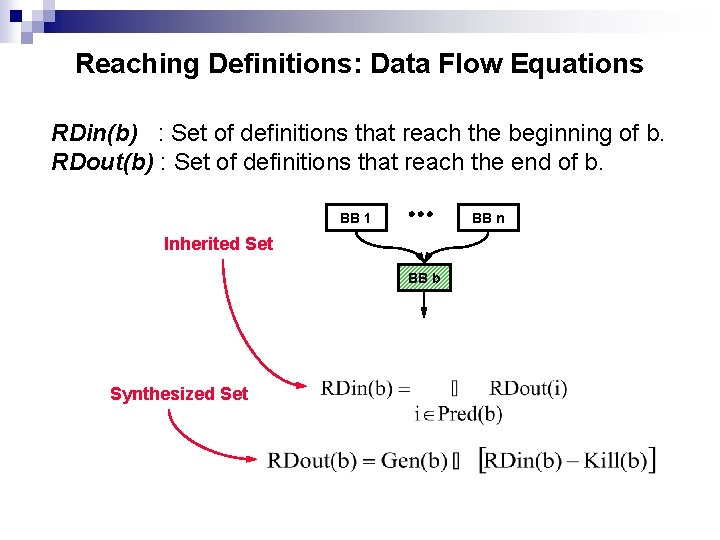Reaching Definitions: Data Flow Equations RDin(b) : Set of definitions that reach the beginning of b. RDout(b) : Set of definitions that reach the end of b. BB 1 BB n Inherited Set BB b Synthesized Set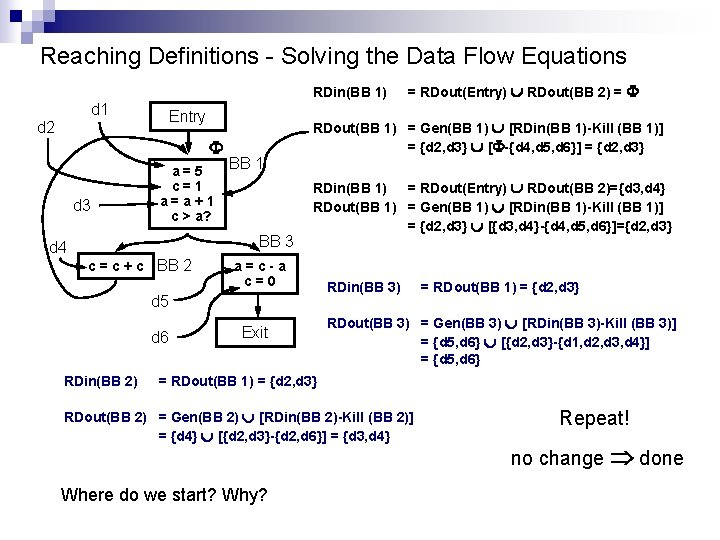Reaching Definitions - Solving the Data Flow Equations RDin(BB 1) d 1 Entry d 2 F d 3 a=5 c=1 a=a+1 c > a? BB 1 BB 3 d 4 c = c + c BB 2 RDout(BB 1) = Gen(BB 1) È [RDin(BB 1)-Kill (BB 1)] = {d 2, d 3} È [F-{d 4, d 5, d 6}] = {d 2, d 3} RDin(BB 1) = RDout(Entry) È RDout(BB 2)={d 3, d 4} RDout(BB 1) = Gen(BB 1) È [RDin(BB 1)-Kill (BB 1)] = {d 2, d 3} È [{d 3, d 4}-{d 4, d 5, d 6}]={d 2, d 3} a=c-a c=0 d 5 d 6 RDin(BB 2) 2) = RDout(Entry) È RDout(BB 2) = F Exit RDin(BB 3) = RDout(BB 1) = {d 2, d 3} RDout(BB 3) = Gen(BB 3) È [RDin(BB 3)-Kill (BB 3)] = {d 5, d 6} È [{d 2, d 3}-{d 1, d 2, d 3, d 4}] = {d 5, d 6} = = RDout(BB 1) 1) = = {d 2, d 3} RDout(BB 2) 2) = = Gen(BB 2) 2) È È [RDin(BB 2)-Kill (BB 2)] = = {d 4} È È [{d 2, d 3}-{d 2, d 6}] = = {d 3, d 4} Where do we start? Why? Repeat! no change Þ done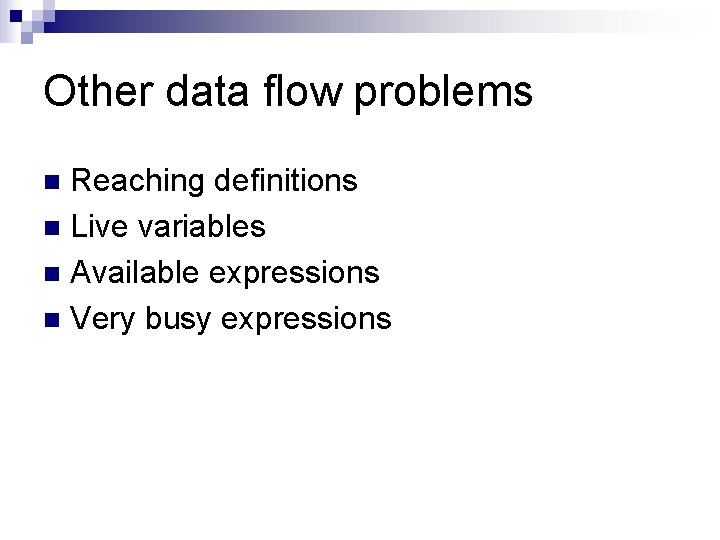Other data flow problems Reaching definitions n Live variables n Available expressions n Very busy expressions n